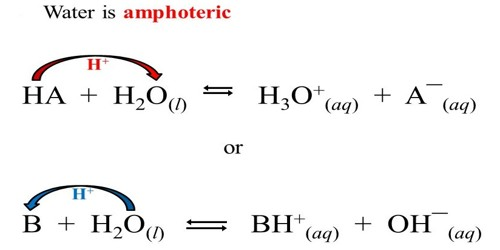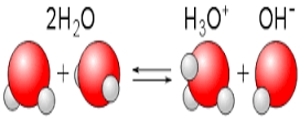Chemistry

# Amphoteric Nature of WaterWater is amphoteric meaning that it can act as both an acid and a base. The water molecule has hydrogen atoms and, for that reason, could act as an acid in a reaction. The amphoteric characteristic of water is important in the acid-base properties of aqueous solutions.

HNO3 (aq) + H2O (I) ↔ NO3 (aq) + H3O+ (aq)

In the forward reaction, HNO3 donates a proton to H2O. Thus, HNO3 is acid and H2O is here a base.In the backward reaction, NO3 accepts a proton from H3O+. Thus, NO3 is acid and H2O is a base.

NH3 (aq) + H2O (I) → NH4+ (aq) + OH (aq)

In this forward reaction, NH3 accepts a proton from H2O. Thus, NH3 is a base and H2O is an acid. In the backward reaction, NH4+ donates a proton to the OH. Here, the OH is a base and NH4+ is an acid. The oxygen atom in the water molecule has two lone pairs, one of which could be used to form a bond with an (H+), and, therefore, the water molecule could act as a base in a reaction. Since water has the potential to act both as an acid and as a base, water is amphoteric.

Amphoteric behavior means the substance can react with both acid and base. According to the “Lowry bronsted concept” – bases stronger than water, lean-to allow proton from it. Thus by donating a proton, water acts as an acid.

H2O + NH3 (a base) → NH4+ + OH  [Bases – accepts a proton]

H2O + HCl (an acid) → H+ + Cl  [Acid – loses a proton (H+)]

Because water can react to both acid and base, we say it is amphoteric. Thus water can donate as well as accept proton and show amphoteric nature.

Explanation:

One of the two water molecules acts as a Bronsted-Lowry acid and donates a proton to the other water molecule, which consequently acts as a Bronsted-Lowry base.

Water can act as an acid or as a base in various chemical reactions, the most common two examples involving the ammonia and hydrochloric acid reactions.

NH3 (aq)+H2O (l) ⇌ NH4+ (aq) + OH (aq)

Here water acts as a Bronsted-Lowry acid because it donates a proton to ammonia.

HCl (g) + H2O (l) ⇌ H3O+ (aq) + Cl (aq)

This time water acts as a Bronsted-Lowry base because it accepts a proton from hydrochloric acid.

To be exact, in reactions that involve the transfer of protons, the term is actually amphiprotic.